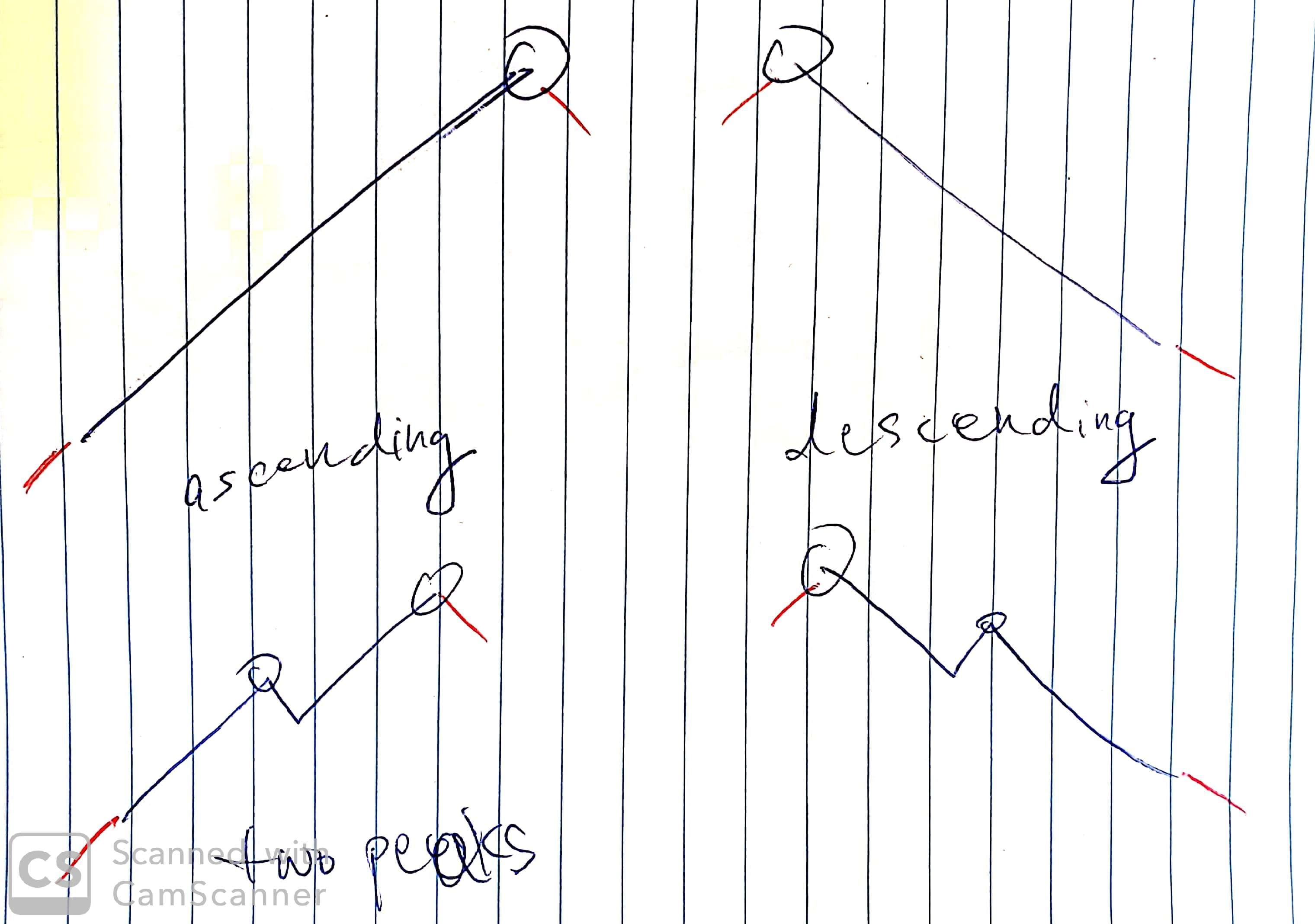# 162. Find Peak Element

Reference: LeetCode
Difficulty: Medium

## Problem

A peak element is an element that is greater than its neighbors.

Given an input array nums, where nums[i] ≠ nums[i+1], find a peak element and return its index.

The array may contain multiple peaks, in that case return the index to any one of the peaks is fine.

You may imagine that nums[-1] = nums[n] = -∞.

Example:

## Analysis

### Brute-Force

Note: Use long type.

Another version in one if:

If we exploit all test cases, we know that the peak must occur in all cases. In other words, the peak is guaranteed.So we just need to check whether there is nums[i] > nums[i + 1] in the array. If not, return the last index as the answer.

The solution version:

Time: $O(N)$
Space: $O(1)$

The reason why we can use binary search for this problem is that when the current trend is ascending or descending we can determine which side to go.

• \ or nums[mid] > nums[mid + 1] or descending: At least one peak lies on the left side, although there is possibly another one on the right side.
• / or nums[mid] < nums[mid + 1] or ascending: At least one peak lies on the right side, although there is possibly another one on the left side.
• Equal case is not possible.

Why do we use lo < hi and hi = mid?

a. lo < hi:

• lo and hi will point to the same element at last.
• If it is lo <= hi, lo will be eventually larger than hi and lo may be out of bound, which is not allowed in this problem.
• Consider the case . If lo <= hi is applied, the code inside the loop will be executed and nums[mid + 1] will be out of bound! So lo < hi makes sure that this won’t happen.

b. hi = mid:

• If / or nums[mid] < nums[mid + 1], we update lo by mid + 1 since the element at mid cannot be the peak.
• If \ or nums[mid] > nums[mid + 1], we update hi by mid instead of mid - 1 since the element at mid could be the peak.

Time: $O(\log{N})$
Space: $O(1)$

CommentJunhao Wang
a software engineering cat